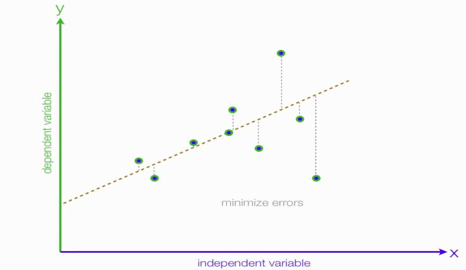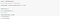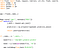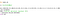# A Glance at Linear Regression and Execute the Linear Regression model as an API Service

Hello everyone! As Machine Learning and Artificial Intelligence is increasingly adopted by a raft of organizations, the demand for ML & AI professionals is increasing at an astonishing rate making it one of the most sought skill in Tech! People across different disciplines are trying to apply AI to make their tasks a lot easier. For example, economists are using AI to predict future market prices to make a profit, doctors use AI to classify whether a tumor is malignant or benign, meteorologists use AI to predict the weather, HR recruiters use AI to check the resume of applicants to verify if the applicant meets the minimum criteria for the job, etc.

For anyone who wants to learn ML algorithms but hasn’t gotten their feet wet yet, you are at the right place. The fundamental algorithm that every Machine Learning enthusiast starts with is a linear regression algorithm. Therefore, I shall do the same as it provides a base for us to build on and learn other ML algorithms.

So, let’s get ourselves accustomed to What Regression is all about!

Linear Regression is a statistical method that allows us to summarize and study relationships between a target variable (also known as dependent variable) on one or more predictors (also known as independent variables).

The objective is to estimate and/or predict the mean value of the dependent variable (continuous) on the basis of the known values of the independent variables. And try to minimize the error as much as possible.Linear Regression

Regression Equation

Some of the examples for Linear regression are:

§ Price of car depends on car weight, fuel efficiency ,manufacturing place and many other features

§ Average hourly wage depends on education and occupational domain

§ Imagine you want to estimate the demand of your customer. Or you want to predict the sales of a particular item in the future.

§ In BPOs/KPOs, we can analyze the relationship between the wait times of caller and the number of complaints

Meaning of Linearity

The term ‘linear’ can be interpreted in two ways:

§ Linearity in the Variables

§ Linearity in the Parameters

Examples of Linear Regression Model

Examples of Non-Linear Regression Model

The term ‘linear’ regression means a regression that is linear in the parameters (β’s). It may or may not be linear in the explanatory variables (X’s).

# Linear Regression: Key Assumptions

Assumption 1: Variability in X feature

Interpretation

§ X values in a given sample must not all be the same.

§ Example: Suppose the modeling data corresponds to a particular year (say, 2018). The ‘year’ variable would take single unique value ‘2018’ for all records. Such a variable won’t add any value in making any prediction.

Conclusion

§ No estimation possible for β coefficient

Assumption 2: Predictor X is non-stochastic

Interpretation

§ Values taken by the regressor X are considered fixed in repeated samples. That is, X is non-random

Conclusion

§ No serious implication as long as predictor X and disturbance e are uncorrelated, which is yet another assumption of Classical Linear Regression Model

Assumption 3: Zero mean value of disturbance e=ε (Random error term)

Interpretation

§ Assumption that E(ei | Xi) = 0 implies that the positive ei values cancel out the negative ei values so that their average or mean effect on Y is zero.

§ E(ei |Xi)=0 also implies that E(Yi |Xi)=β0+β1X1 +…+βkXk (given that Yi =β0+β1X1 +…+βkXk +ei ).

Conclusion

§ No impact on the properties of slope coefficients (β1, β2, …, βk).

§ If E( (ei | Xi) is a non-zero constant, we get a biased estimate of intercept β0

Assumption 4: Homoscedasticity

Interpretation

§ Given the value of X, the variance of disturbances ei is the same for all observations

Variance (ei | Xi)= σ 2

Conclusion

§ Absence of homoscedasticity implies presence of heteroscedasticity. OLS estimates remain unbiased.

§ But OLS estimates no longer remain efficient (i.e. there are alternative methods of estimation such as WLS with smaller standard errors) and hence significance tests may not be valid.

Assumption 5: No autocorrelation between disturbances (e=ε)

Interpretation

§ Given any two X values, Xi and Xj (i ≠ j), the correlation between ei and ej (i ≠ j) is zero.

§ This assumption is more likely to get violated in case of time-series data. Usually, generalized least square (GLS) models are used to tackle this problem.

Conclusion

§ OLS estimates remain unbiased.

§ But OLS estimates no longer remain efficient and hence significance tests may not be valid.

Assumption 6: Zero covariance between ε and X

Interpretation

§ X and e are assumed to be uncorrelated, as the definition of PRF requires that X and e have separate (and additive) influence on Y

Conclusion

§ OLS estimates not only become biased, but also inconsistent (i.e. as the sample size increases indefinitely, the estimators do no converge to their true population values)

Assumption 7: n > k + 1

Interpretation

§ Number of observations (n) must be greater than the number of parameters to be estimated (k + 1) where k = Number of Independent Variables (X1, X2, …, Xk)Parameters to be estimated include k slope coefficients (β1, β2, …, βk) plus 1 intercept coefficient (β0)

Conclusion

§ Regression coefficients can’t be estimated

Assumption 8: No perfect multicollinearity ( Inter-correlation analysis and VIF test are popular methods of detecting multicollinearity)

Interpretation

§ There are no perfect linear relationships among the explanatory variables

Conclusion

§ Perfect Multicollinearity Case

− Coefficients are indeterminate and standard errors are not defined

§ High Multicollinearity Case

− Estimation of regression coefficients is possible, but standard errors tend to be large

− Individual variable contribution tends to be less precise as predictors are highly correlated

− Multicollinearity leads to model over-fitting. The overall measure of goodness of fit can be very high, but the t-ratio of one or more variables may be statistically insignificant.

Assumption 9: Normality of ε

Interpretation

§ εi follow the normal distribution

Conclusion

§ Estimates remain BLUE (An OLS estimator βˆi is said to be Best Linear Unbiased Estimator (BLUE) of βi)

§ But they are no longer asymptotically efficient (i.e. as sample size grows, estimates are not optimal)

# Some of the well know Feature Selection /Reduction Methods

FORWARD (i.e. Forward Selection)

This technique begins with no variable in the model and then the variables are added one by one to the model based on their F statistics

− For each independent variable, F statistics are computed (reflecting variable contribution to the model)

− Variable with the largest F statistic is added to the model if its p-value < defined criteria

− Process is repeated until there is no independent variable whose F statistic is more significant than defined criteria

− Once a variable is in the model, it stays

BACKWARD (i.e. Backward Elimination)

This technique begins with all variables in the model and then the variables are deleted one by one from the model based on their F statistics

− For each independent variable, F statistics are computed (reflecting variable contribution to the model)

− Variable with the smallest F statistic is deleted from the model if its p-value > defined criteria

− Process is repeated until all the variables in the model produce F statistic significant at defined criteria

− Once a variable is removed from the model, it is never re-considered for inclusion

STEPWISE (i.e. Stepwise Elimination)

This technique is similar to the FORWARD selection technique except that the variables already in the model do not necessarily stay there

− Variables are added one by one to the model and the F statistic for a variable to be added must be significant at the defined criteria

− Once a variable is added, stepwise method looks at all the variables in the model and deletes any variable that does not produce an F statistic significant at defined criteria

− Variables are thus entered into and removed from the model in such a way that each forward selection step may be followed by one or more backward elimination steps

− Stepwise process terminates

# Linear Regression Performance Measures

Some of the performance measure are listed below.

§ MAPE

§ R2 (R-Square)

§ Adjusted R2 (R-Square)

§ Root Mean Squared Error (RMSE)

§ Coefficient of Variation (COV)

§ Residual Analysis

Mean absolute percentage error (MAPE) :- It is a statistical measure of how accurate a prediction system is. It measures this accuracy as a percentage, and can be calculated as the average absolute percent error for each time period minus actual values divided by actual values

n= Number of fitted points

y` =Predicted values

y = Actual values

R2 (Coefficient of Determination)

k-Variable Linear Regression Equation

Observed: Y= β0+ β1X1+…+ β’kXk +e

Model : Y= β0+ β1X1+…+ βkXk

R2 Interpretation

Proportion of variation in target variable ( Y ) explained by the model ( Yˆ )

R2 is a goodness-of-fit measure, which is also known as coefficient of determination

R2 Definition 1

R2 = ESS / TSS =1− RSS / TSS

ESS = ∑ (Y Y `) ^2 = Explained sum of square (Also known as Regression sum of Squares

RSS = (Y Y `) ^2 = Residual Sum of Squares

TSS = (Y Y `)= Total Sum of Squares = ESS + RSS

R2 Definition 2

R2 = (correlation(Y,Y))^2

Adjusted R2

Adjusted R2 is a modification of R2 that adjusts for the number of explanatory terms in the model

§ Unlike R2, adjusted R2 increases only if the new term improves the model more than expected by chance

§ Adjusted R2 can be negative

§ Adjusted R2 < R2

Where

R2 =UnadjustedR-Square

n = Number of observations in the sample
k = Number of explanatory variables
m = 1 if model has an intercept term; otherwise m = 0

(Higher R2 and Adjusted R2 values indicate better model performance)

Root Mean Squared Error (RMSE)

§ Estimate of standard deviation of the error term.

§ Calculated as square root of Mean Squared Error (MSE).

§ Scale dependent metric which does not have standalone meaning.

§ Used for comparison across models for model selection.

where,

Y =Observed value

Y ` =Predicted value

n = Number of observations

Coefficient of Variation (COV)

§ COV is calculated as ratio of RMSE to Dependent Variable Mean, multiplied by 100.

§ Unlike RMSE, it is a unit-less expression of variation in data

where

RMSE = Root Mean Squared Error

Y ` =Average Value of Dependent Variable

# Interpreting residual plots to improve your Regression

Need for Residual Analysis

Objective 1: To check whether the residuals are ‘pattern less’ (randomly scattered) centered around zero Method of Analysis: Residual Plot

Objective 2: To check whether the residuals follow a normal distribution Method of Analysis: Normal Q-Q Plot

Residual Plot

§ A graph that shows the residuals on the vertical axis and the fitted values on the horizontal axis.

§ If the points in a residual plot are randomly dispersed around zero (horizontal axis), a linear regression model is appropriate for the data, otherwise a non-linear model is more appropriate.

Normal Q-Q Plot

§ Quantile-Quantile (Q-Q) plot is a graphical method for comparing two probability distributions by plotting their quantiles against each other.

§ Normal Q-Q plot shows the observed quantiles of residuals on the vertical axis and the theoretical quantiles of standard normal distribution on the horizontal axis.

§ If residuals follow normal distribution, the normal Q-Q plot should be a straight line

These are some of the concepts for multiple regression I’ve mentioned. The second part of this article I will walk you through on How we can Execute the Linear regression Model.

# Linear Regression model as an API Service

As a data scientist, Our primary task is to make an impact with our machine learning models. When we start a new project, our primary task is to understand the business requirement, explore the data sources and the key decisions on the technology stacks.

You start exploring the data and build hypotheses around it. Once you’ve got a full understanding of what data you’re dealing with and have aligned with the client on what steps to be taken, one of the outcomes can be to create a predictive model.

The process of building a predictive model is iterative until the results are presented and everyone is happy. In order to create an impact this model needs to be executed within client infrastructure. Also, when the model is used by other people you can get the necessary input to improve the model and each step. But how quickly we can do this also depends on complication of infrastructure and how familiar we are with it!

So in-order to tackle this situation, you need a tool that can fit in the complicated infrastructure, preferably in a language that you’re familiar with. This is where you can use one of the tool known as Flask. Flask is fun and easy to setup. This micro framework for Python offers a powerful way of annotating Python function with REST endpoint. I’m using Flask to publish ML model API that allows you to send data, and receive a prediction as a response.

For better code maintenance, I would recommend using a separate Jupyter notebook where we will build a simple ML model. The objective of this article is to Execute your model as an API service. So we will not much emphasis on Data cleaning, Feature engineering, Hyper parameter tuning or other core modeling activities.

For the purpose of demonstration, I will train a simple Linear Regression model to predict the Salary of NBA Player using a Kaggle dataset.

# To access code and dataset please clone the Git Repo. The csv file has all the required data for this Analysis.

Import all the necessary libraries

If the libraries are not available on your system please use :

pip install <Library name>

Import the Dataset and have look at couple of rows

Our data is clean and we are assuming that all the data preparation activities are already taken care off , So we directly jump into Model building and Execution task.

Split the dataset into Train and test

Separate the Target and Predictor features

Let’s Build a simple Linear Regression model and generate the pickle for it.

It is always a good practice to do a test run and check if the model performs well. Construct data frame with an array of column names and an array of data (using new data, the one which is not present in train or test datasets).

Compare the train and test dataset score, they seems to be close enough.Regression Model

Once the model you have created seems to perform well, you can save it as file. You can then open this pickle file later and call the function Predict to get a prediction for new input data. This is exactly what we will do in Flask.Pickle file

Develop a Flask Api service and Execute it

Flask runs on a server. This can be in the environment of the client or a different server depending on the client’s requirements. When we are running python flask_app.py , it will first loads the created pickle file. Once this is loaded you can start making predictions.

While it works to start Flask interface directly in Jupyter notebook, I would recommend to convert it to Python script and run from command line as a service.

Python script with Flask endpoint can be started as the background process. This allows to run endpoint as a service and start other processes on different ports.Flask_app.py

Request predictions

Predictions are made by passing a POST JSON request to the created Flask web server which is on port 5000 by default. In flask_app.py this request is received and a prediction is based on the already loaded prediction function of our model. It returns the prediction in JSON format.request.py

Now, all you need to do is call the web server with the correct syntax of data points. The input dataset should have the same data format and shape on which the pickle file was prepared, to get this JSON response of your predictions.

For example, the below output:

`python request.py -> <Response > "[2566.74726972]"`

For the data we sent we got a prediction of Salary “`2566.74726972`” as output of our model. Basically, in the above steps we are just sending an array of data to an endpoint. This will transform the data to a Json format. The endpoint reads the JSON post and transforms it back to the original array.

This simple approach can easily let other people use your machine learning model and quickly make an impact.

Feel free to improvise on this approach. Suggestion are always welcome.

Full code:

https://github.com/himswamy/ml-flask-api.git

# Happy Learning!

## The Startup

Get smarter at building your thing. Join The Startup’s +724K followers.

Written by

## Himanshu Swamy

Data Scientist |Machine Learning Engineer| Deep Learner | NLP

## The Startup

Get smarter at building your thing. Follow to join The Startup’s +8 million monthly readers & +724K followers.

Written by

## Himanshu Swamy

Data Scientist |Machine Learning Engineer| Deep Learner | NLP## The Startup

Get smarter at building your thing. Follow to join The Startup’s +8 million monthly readers & +724K followers.

Medium is an open platform where 170 million readers come to find insightful and dynamic thinking. Here, expert and undiscovered voices alike dive into the heart of any topic and bring new ideas to the surface. Learn more

Follow the writers, publications, and topics that matter to you, and you’ll see them on your homepage and in your inbox. Explore

If you have a story to tell, knowledge to share, or a perspective to offer — welcome home. It’s easy and free to post your thinking on any topic. Write on Medium